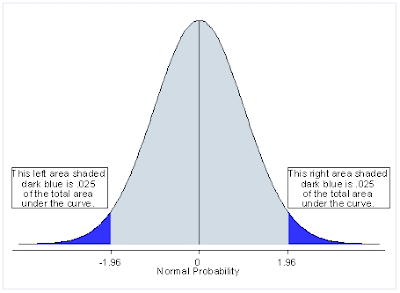« Go Back To Previous Page

# Oh, it’s the notorious “p-value” again! What does the p-value tell us?

Published on May 16th, 2013 under topic "General" by KISS Consulting

A common error made when interpreting statistical results is linking statistical significance with practical importance. We can’t emphasize strongly enough that statistical significance does not equate to practical importance, and likewise practical importance does not guarantee statistical significance. Often, we witness students or even experienced researchers being obsessed with obtaining small p-values in hopes of giving their study a “wow” conclusion. There are two divisions in statistics: descriptive statistics and inferential statistics.

Descriptive statistics is a presentation of the characteristics of datasets, including the mean, standard deviation and the frequency distribution of values.

Inferential statistics, which is more advanced than descriptive statistics, is used to make inference to a wider population based on a smaller sample. For example, we often hear the predicted voting results of an election provided by the media. Since it is not possible to ask every eligible voter which party they would vote for, we can only project the voting results of the whole nation by collecting a sufficient sample of voters Research does not exist in a vacuum. We cannot be 100% absolutely sure about our research conclusion.  There is always a chance, even a tiny slight one, that we might be wrong. The sample we collect won’t be a perfect representation of the bigger targeted population. Why? It is simply because that the collected sample does not cover the whole population.  We logistically (as well as financially) are not able to sample everyone, so we need to draw inferences about the population from our sample.

So, now the stage is set up for our big star tonight- the p-value! Everyone who attended statistics class must have heard about Type I and Type II error.  We are bound to make certain errors in the process of hypothesis testing. The p-value (which stands for probability value by the way) is the probability of your test statistic being at least as high as the one you obtained, assuming that the null hypothesis is true. So the p-value is telling us how close our data matches that of the null hypothesis. A high p-value means that we have a higher probability of it being consistent with the null hypothesis and a low p-value means that we have a lower probability of it being consistent with the null hypothesis.

The convention for p-values is to have a cut-point of <=0.05 as that denoting statistical significance.

Why is the p-value the basis for interpreting statistical results?

Well, let’s look at a hypothetical example.

A social scientist would like to examine if adults who are more active in doing volunteer work are more likely to vote.  The null hypothesis is that the likelihood of voting for an adult who puts in more than 2 hours of volunteer work every week is as the same as that of his or her counterpart who participated in volunteer work for less than 2 hours weekly.

Now this researcher needs to show that any discrepancy that exists between the two groups in voting behavior is due only to the hours of volunteering work.  In this case, other factors, (i.e. education, income, ethnicity, geographic location, family type, age, gender) should be treated as control factors in the statistical models.

Say, this researcher did a perfect job of using the right statistical model to analyze the data. Now how does p-value in the data output support or disagree with the research argument?

The commonly-used standard for statistical significance in social sciences is p<=.05. However, to reduce the possibility of making a Type I error, you may see p<.01 or p<.001 used.

To continue with our previous example: if the statistical results indicate the p-value is .049, would that be sufficient to jump to the conclusion that doing volunteer work for 2 hours every week is a strong predictor of voting behavior?

Well, yes and no.

Yes because we can say volunteering is a statistically significant factor.

No because we cannot say it is a strong predictor as the strength of the predicting variable is decided by the magnitude of its effect size and not just the p-value

Take home messages for statistical significance and p-values:

1. Statistical significance must be taken in connection with the existing literature in your field. The results do not stand alone by themselves. You need to have a well-established conceptual framework guiding the development of your hypothesis and the interpretation of statistical significant findings.
2. Statistical significance should not be the only goal of your research. Bear in mind the picture of a forest instead of single tree. Statistical significance should not be interpreted as practical significance, importance or meaningfulness.
3. The p-value does NOT tell you that your results are due to chance. This is a common misconception. It can tell us that the pattern of data we observed is consistent with what could occur by chance.
4. If our p-value is >0.05 we do NOT accept the null hypothesis. We don’t know the true state of the null hypothesis, only that our data has failed to reject the null hypothesis in this case.